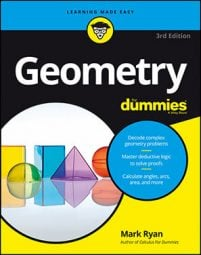##### Geometry For DummiesA rotation is a transformation in which the pre-image figure rotates or spins to the location of the image figure. With all rotations, there's a single fixed point—called the center of rotation—around which everything else rotates.

This point can be inside the figure, in which case the figure stays where it is and just spins. Or the point can be outside the figure, in which case the figure moves along a circular arc (like an orbit) around the center of rotation. The amount of turning is called the rotation angle.

The easiest way to understand this is by doing a problem: In the following figure, pre-image triangle ABC has been rotated to create image triangle A'B'C'. Find the center of rotation.

To begin, take the three segments that connect pre-image points to their image points (in this case, line AA', line BB', and line CC'). In all rotations, the center of rotation lies at the intersection of the perpendicular bisectors of such segments. Because the three perpendicular bisectors meet at the same point, you need only two of them to find the point of intersection. Any two will work, so find the perpendicular bisectors of lines AA' and BB'; then you can set their equations equal to each other to find where they intersect.

First get the midpoint of line AA':

Then find the slope of line AA':

The slope of the perpendicular bisector of line AA' is the opposite reciprocal of –9/2, namely 2/9. The point-slope form for the perpendicular bisector is thus

Go through the same process to get the perpendicular bisector of line BB':

The slope of the perpendicular bisector of line BB' is the opposite reciprocal of –9/5, which is 5/9. The equation of the perpendicular bisector is thus

Now, to find where the two perpendicular bisectors intersect, set the right sides of their equations equal to each other and solve for x:

Multiply both sides by 9 to get rid of the fractions; then divide:

Plug –10 back into either equation to get y:

You've done it. The center of rotation is

Give this point a name—how about point Z?

The following figure shows point Z, angle AZA', and a little counterclockwise arrow that indicates the rotational motion that would move triangle ABC to triangle A'B'C'. If you hold Z where it is and rotate the image counterclockwise, triangle ABC will spin to where triangle A'B'C' is now.